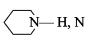The ionisation energy will be maximum for the process:

(a) $Ba\to B{a}^{2+}$

(b) $Be\to B{e}^{2+}$

(c) $Cs\to C{s}^{+}$

(d) $Li\to L{i}^{+}$

Concept Questions :-

Ionic Bonding
High Yielding Test Series + Question Bank - NEET 2020

Difficulty Level:

In which of the process, the bond order increases and magnetic behaviour changes?

(a) ${N}_{2}\to {{N}_{2}}^{+}$

(b) ${C}_{2}\to {{C}_{2}}^{+}$

(c) $NO\to N{O}^{+}$

(d) ${O}_{2}\to {{O}_{2}}^{+}$

Concept Questions :-

M.O.T
High Yielding Test Series + Question Bank - NEET 2020

Difficulty Level:

In piperidineatom has hybridization:

(a) sp

(b) $s{p}^{2}$

(c) $s{p}^{3}$

(d) $ds{p}^{2}$

Concept Questions :-

Hybridisation
High Yielding Test Series + Question Bank - NEET 2020

Difficulty Level:

Specify the coordination geometry around and hybridization of N and B atoms in a 1:1 complex of $B{F}_{3}$ and $N{H}_{3}$:

(a) N: tetrahedral, $s{p}^{3}$; B : tetrahedral, $s{p}^{3}$

(b) N: pyramidal, $s{p}^{3}$; B : pyramidal, $s{p}^{3}$

(c) N: pyramidal, $s{p}^{3}$; B: planar, $s{p}^{2}$

(d) N: pyramidal, $s{p}^{3}$; B: tetrahedral, $s{p}^{3}$

High Yielding Test Series + Question Bank - NEET 2020

Difficulty Level:

Assuming $2s-2p$ mixing is NOT operative, the paramagnetic species among the following is :

(a) $B{e}_{2}$

(b) ${B}_{2}$

(c) ${C}_{2}$

(d) ${N}_{2}$

High Yielding Test Series + Question Bank - NEET 2020

Difficulty Level:

When ${O}_{2}$ is adsorbed on a metallic surface, electron transfer occurs from the ,metal to ${O}_{2}$. The wrong statements(s) regarding this adsorption is (are):

(a) ${O}_{2}$ is physisorbed

(b) Heat is released

(c) Occupancy of $\pi *2p$ of ${O}_{2}$ is increased

(d) Bond length of ${O}_{2}$ is increased

Concept Questions :-

Covalent Bonding
High Yielding Test Series + Question Bank - NEET 2020

Difficulty Level:

Born Haber cycle is used to determine:

(a) lattice energy

(b) electron affinity

(c) ionization energy

(d) either of them

Concept Questions :-

Covalent Bonding
High Yielding Test Series + Question Bank - NEET 2020

Difficulty Level:

Select the most ionic and most covalent compounds respectively from the following.

(A)                                 (B)

(C)                                  (D)

Concept Questions :-

Acid, Base and Fajan's Rule
High Yielding Test Series + Question Bank - NEET 2020

Difficulty Level:

The hybridization of atomic orbitals of nitrogen in  are :

(A)                                   (B)

(C)                                  (D)

Concept Questions :-

Hybridisation
High Yielding Test Series + Question Bank - NEET 2020

Difficulty Level:

In which of the following sets the central atom of each member involves $s{p}^{3}$ hybridisation?

(A)                                       (B)

(C)                                     (D)

Concept Questions :-

Hybridisation